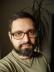## Rotate Matrix

Write a script to rotate the following matrix by given 90/180/270 degrees clockwise.
```[ 1, 2, 3 ]
[ 4, 5, 6 ]
[ 7, 8, 9 ]```

For example, if you rotate by 90 degrees then expected result should be like below

```[ 7, 4, 1 ]
[ 8, 5, 2 ]
[ 9, 6, 3 ]```

The easiest way to work with multidimensional data in Perl is PDL. Interestingly, I haven’t found a direct method to rotate a matrix in this way.

What I have found, though, was a method to transpose a matrix, which means to switch the columns and rows. The result for the sample input is

```[1, 4, 7],
[2, 5 ,8],
[3, 6, 9]```

Unfortunately, it’s not what we want. We need to reverse each line of the result. This can be achieved by calling the `slice` method with the argument of `[-1, 0]` which means "return the elements of the first dimension from the last one to the first one”.

``````#!/usr/bin/perl
use warnings;
use strict;

use PDL;

my \$matrix = pdl([1, 2, 3],
[4, 5, 6],
[7, 8, 9]);

for my \$rotation (0, 90, 180, 270) {
my \$times = \$rotation / 90;
my \$result = \$matrix;
\$result = \$result->transpose->slice([-1, 0]) for 1 .. \$times;
print "\$rotation:\$result";
}``````

## Vowel Strings

Write a script to accept an integer 1 <= N <= 5 that would print all possible strings of size N formed by using only vowels (a, e, i, o, u). The string should follow the following rules:
• a’ can only be followed by ‘e’ and ‘i’.
• e’ can only be followed by ‘i’.
• i’ can only be followed by ‘a’, ‘e’, ‘o’, and ‘u’.
• o’ can only be followed by ‘a’ and ‘u’.
• u’ can only be followed by ‘o’ and ‘e’.

For example, if the given integer N = 2 then script should print the following strings:

```ae
ai
ei
ia
io
iu
ie
oa
ou
uo
ue```

A recursive solution similar to last week’s Stepping Numbers came to my mind, but I decided to solve this task differently.

Let’s see how we can replace recursion with a loop. We need to keep an array with the strings to process; each iteration of the loop will generate a new array of extended strings that will be used in the next iteration (or as the result in the last iteration).

``````#!/usr/bin/perl
use warnings;
use strict;
use feature qw{ say };

my %next = (
a => [qw[ e i ]],
e => [qw[ i ]],
i => [qw[ a e o u ]],
o => [qw[ a u ]],
u => [qw[ o e ]]);

my \$n = shift;
die "Invalid argument" unless (\$n // "") =~ /^[1-5]\$/;

my @agenda = sort keys %next;

while (\$n > length \$agenda) {
my @next;
for my \$string (@agenda) {
my \$last = substr \$string, -1;
push @next, map \$string . \$_, @{ \$next{\$last} };
}
@agenda = @next;
}
say for @agenda;``````I blog about Perl.